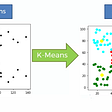# What is Ensemble Learning?

Ensemble Learning is a technique or process in which multiple models are generated and combined to solve a particular machine learning problem. You can think of them as meta-algorithms that combine multiple models together primarily to improve the performance of a model and reduce the variance of the outcome.

Choosing which model to use is extremely important in any regression or classification problem and the choice depends on many variables such as the quantity of data, distribution of data, and its types.

If we look at supervised machine learning, an algorithm creates a model from training data with the goal to best estimate the output variable (y) given the data (X). To predict this output variable with a high degree of accuracy we need to understand the bias-variance trade-off of specific algorithms in regards to the training data. The bias-variance trade-offs of these algorithms (base-models) will tell us what type of ensemble learning methods to perform, as some methods will be used to increase bias while others to decrease variance. So, before we get into different ensemble methods I want to go through what bias and variance mean to understand how their trade-offs will affect the methods we choose.

Bias and variance are the two most fundamental features in a model, the idea is that we tune our parameters in such a way that our bias and variance balance out to create good predictive performances. Different types of data need different types of algorithms and with these unique algorithms come varied trade-offs. Another way of looking at this trade-off is that we want our models to have enough degree of flexibility to resolve any underlying complexity of new datasets but not so much that the model has less accuracy on the training and test data.

## Bias Error

Bias errors are assumptions made by a model to make the target easier to predict or in other words, it is how close our average predictions are to the actual correct values. The lower the bias Error the more accurate our predictions will be on our train and testing data, the higher the bias errors the less accurate our predictions will be. (Bias is the ability to approximate data).

• Low Bias means fewer assumptions about the form of the target function.
• High Bias means more assumptions about the form of the target function or in other words, High Bias means the model pays little attention to the training data and oversimplifies the model. This will lead to high errors in the training and test data. Linear Regression model would have a high bias if trying to fit a quadratic relationship.

## Variance Error

Variance error is the amount that the estimate of the target function will change if the different training data was used. We should expect the algorithm to have some variance no matter what and if we introduce new training sets we should expect a change, but ideally, we do not want to see too much variance from one set to the next. If we do not see much change, we know that the base model is good at picking out hidden underlying mappings between the input and the output variables.

Algorithms with high variance are strongly influenced by the training data set and will not generalize well when it comes to new data sets. Algorithms with a lot of flexibility also have a high variance, these tend to be nonlinear algorithms like Decision Trees or K-Nearest Neighbors, While linear algorithms tend to have low variance. (variance shows the stability of the model).

• Low Variance suggest small changes to the estimate of the target function
• High variance suggests large changes to the estimate of the target function. High variance models will pay a lot of attention to training data and do not generalize well on the data which it has not seen before.

Low Bias Algorithms: Trees, K-Nearest Neighbors and Support Vector Machines

High Bias Algorithms: Linear Regression, Linear Discriminant Analysis, and Logistic Regression.

Low-Variance Algorithms: Linear Regression, Linear Discriminant Analysis, and Logistic Regression

High-Variance Algorithms: Decision Trees, K- Nearest Neighbors, and Support Vector Machines.

The whole goal is to achieve a low-bias and low-variance. Ex. Linear algorithms have low variance and high bias, while nonlinear algorithms have high variance and low bias, in turn, both will need different ensemble approaches to make the prediction value more accurate. These different ensemble approaches will help to improve the error results by combining several models.

## Bagging:

Bagging trains each model in the ensemble using a randomly chosen subset of the training data. By training our models on randomly chosen subsets we can get a good generalized idea of the distribution in our original set and thus create strong predictions. Bagging uses a parallel ensemble method, training each model independently from each other on randomly chosen subsets. An example of this would be the Random Forest Algorithm. Bagging often aims to reduce Variance.

## Boosting:

Boosting is a sequential ensemble method that combines weak learning into strong learners. It aggregates together multiple models, each model compensating for the weakness of the last. Boosting will take a weak model like the regression or tree based-model and improve it. For example, XGBoost is a decision tree based algorithm that uses gradient boosting to improve itself, with its main focus in reducing bias by putting efforts on the most difficult observations.

Popular Boosting techniques:

1. AdaBoost: AdaBoost specifically designed for classification problems. when training a decision tree, it starts by training the observations and assigning each an equal weight. After evaluation of the first set, it will increase the weight of those that are difficult to classify and lower the easy ones. It will iterate these processes until and good predictive value is the outcome.
1. Gradient Boost: Rather than focusing on the data points, gradient boost performs the same method of adjusting weights to the loss functions by using its vector gradient. The loss function is a measure indicating how well off the models coefficient is at fitting the underlying data (The difference from actual to predicted). Gradient Boost works well with both classification and regression.

## Stacking:

Stacking combines multiple classifications or regression models with a meta-classifier or meta-regressor. The base models are trained on the complete training set and the meta-models are trained on the outputs of the base level models as features.

Key Points:

• Ensemble Learning is a technique or process in which multiple models are generated and combined to solve a particular machine learning problem.
• Choosing which model to use is extremely important in any regression or classification problem and the choice depends on many variables such as the quantity of data, distribution of data, and its types.
• Bias and variance are the two most fundamental features in a model, the idea is that we tune our parameters in such a way that our bias and variance balance out to create good predictive performances.
• Different types of data need different types of algorithms and with these unique algorithms come varied trade-offs.
• Linear algorithms have low variance and high bias, while nonlinear algorithms have high variance and low bias.
• Bagging trains each model in the ensemble using a randomly chosen subset of the training data.
• Bagging often aims to reduce Variance.
• Boosting is a sequential ensemble method that combines weak learning into strong learners. It aggregates together multiple models, each model compensating for the weakness of the last.
• Boosting often aims to reduce Bias.

To understand which method to choose from, it is important to understand what type of data we are working with and to understand the short-comings of different regression and classification models. Once we understand the bias and variance trade-offs from these models we can begin to select an ensemble method that will give us the best possible outcome.

--

--

--

## More from Analytics Vidhya

Analytics Vidhya is a community of Analytics and Data Science professionals. We are building the next-gen data science ecosystem https://www.analyticsvidhya.com

## Building a Deep Learning Person Classifier## Reinforcement Learning Explained Visually (Part 4): Q Learning, step-by-step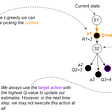## Machine Learning## Natural Language Processing## ComBox x64 Movidius PCIe board for neural networks inference## Computer Vision: Face Mask Detection with CNN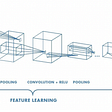## Sklearn from beggining(Part 3) Model Training and performance Analysis## Cross validation in Data Science## NumPy for Data Science Interviews: Part 02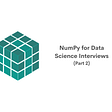## ML Algorithms from scratch in Python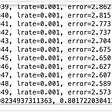## K-Means: The maths behind it, how it works and an example